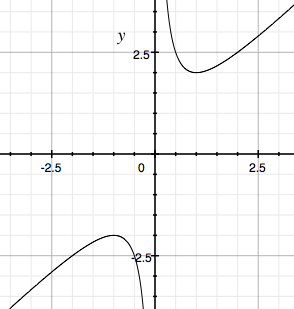SEARCH HOMEMath Central Quandaries & QueriesQuestion from kemelo, a student: show for the following function f(x)=x+1/x has its min value greater than its max valueHi,

The maximum and minimum are relative extrema.

To understand the problem I would sketch a diagram. You probably have an app on your computer that will graph the function. Here is what Grapher produced on my Mac.Graph of y = x + 1/x

You can see that the graph indicates a relative minimum near $x = 1,$ a relative maximum near $x = -1$ and the relative maximum is less than the relative minimum. Can you use calculus now to verify that this is true?

PennyMath Central is supported by the University of Regina and the Imperial Oil Foundation.